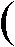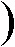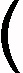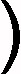edHelper subscribers - Create a new printable

Sample edHelper.com - Matrices Worksheet

 Name _____________________________Date ___________________
Matrices
Complete.
1.15 -6 17 13 16 -7+-15 -11 -4 -14 17 112.19 13+17 20Complete.
3.15 -17 -6 1 -10 11 7 -2 -19 19 -15 4--7 2 -1 3 -14 -17 5 14 17 -11 13 94.-7 -20 18-17 -11 6Complete.
5.4 -3+-20 -18-18 9Complete.
6.-18 17 2 0 -12 -9 -8 11 -15 8 10 6-4 12 18 -1 13 5 14 -4 -5 -2 -19 9--7 -10 -13 11 -15 -2 -6 3 4 12 16 8Find the product. If the product is not defined, state the reason.
7.11 -1 19 12 6 -2 2 3 10 9 0 -16 -6 18 13 -4-17 0 -14 6 -2 19 10 -6 16 -7 14 8 15 -16 -13 -9Solve for x and y.
8.x 8 16 -1917 -15 14 -3=-228 276 y -183Calculate the determinant of each matrix. Show your work.
9.18 5 9 -410.-6 18 -17 1611.-9 5 16 -16Calculate the determinant of each matrix. Show your work.
12.   Use the diagonals method.0 14 -6 1 -11 -13 -20 15 1113.   Expand along column 1.-8 17 -14 -17 -6 9 8 2 -914.11 -19 18 -11Use determinants to calculate the area. Show your work.
 15 Calculate the area of the triangle with the following points:(0, -4), (2, -2), (-4, -2)
 16 Calculate the area of the triangle with the following points:(-6, 1), (0, 0), (-2, 3)
 17 Calculate the area of the triangle with the following points:(0, -5), (-1, -9), (5, -1)

Use determinants to calculate the area. Show your work.
 18 Calculate the area of the quadrilateral with the following points:(-1, -3), (0, 0), (2, 1), (4, -7)
 19 Calculate the area of the quadrilateral with the following points:(-1, 3), (-1, 8), (3, 5), (7, -1)
 20 Calculate the area of the quadrilateral with the following points:(-4, -2), (-2, 1), (1, 0), (5, -3)

Complete.
21.   Find the inverse of:8 -3 -5 -822.   Find the inverse of:7 -5 4 -1023.   Find the inverse of:6 -6 -4 12Solve the matrix equation.
24.9 -10 12 0X    =62 59 -24 1225.-6 -2 5 -8X    =-84 60 -17 -79Write the system of linear equations represented by each matrix equation.
26.8 -4 3 2x y=148 2427.-9 -6 5 -8 -9 -4 5 -1 -7x y z=28 55 -3Use the inverse of the linear system to solve for x, y, and z.
28.   -3x + 8y + 1z = -18
-2x - 6y - 9z = -15
-6x + 5y + 3z = 13
A-1  =27/353 -19/353 -66/353 60/353 -3/353 -29/353 -46/353 -33/353 34/35329.   9x - 8y + 4z = 52
-1x - 7y - 2z = 13
-2x + 8y + 4z = -52
A-1  =3/65 -16/65 -11/65 -2/65 -11/65 -7/130 11/130 14/65 71/260Write the augmented matrix for the linear system and then solve.
 30 -6x - 7y = -123x + 2y = 15
 31 -1x + 3y = -145x - 6y = 25

Solve each system of equations using Cramer's rule.
 32 -6x - 4y + 7z = -1321x - 6y + 3z = -13-4x - 7y - 9z = 53
 33 5x - 2y = -7-4x + 5y = -8
 34 -3x + 7y = 65-9x + 6y = -15

Sample
This is only a sample worksheet.

edHelper subscribers - Create a new printable